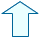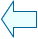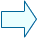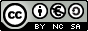The subtraction of natural numbers Operations with natural numbers I

 1. The subtraction of natural numbers. Terms of the subtraction. -Subtraction is the opposite operation of addition. -When you subtract, you take the value of the smaller number from the value of the larger one. -You also subtract when you want to know how much you must add to the smaller number so that it becomes the same as the larger number. The terms of subtraction are called minuend and subtrahend, the outcome is called the difference. The minuend is the first number, it is the number from which you take something and it must be the larger number. The subtrahend is the number that is subtracted and it must be the smaller number. The difference is the result of the subtraction. Esta unidad interactiva requiere la máquina virtual de Java J2RE. To check if the subtraction is correct we add up the subtrahend and the difference. The outcome must be the minuend.Eduardo Barbero CorralSpanish Ministry of Education. Year 2007Except where otherwise noted, this work is licensed under a Creative Common License